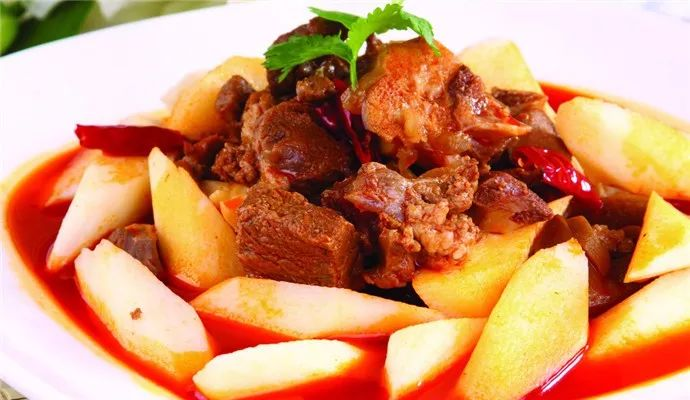32-t}function r(e,r,i,s,o,u){return t(n(t(t(r,e),t(s,u)),o),i)}function i(e,t,n,i,s,o,u){return r(t&n~t&i,e,t,s,o,u)}function s(e,t,n,i,s,o,u){return r(t&in&~i,e,t,s,o,u)}function o(e,t,n,i,s,o,u){return r(t^n^i,e,t,s,o,u)}function u(e,t,n,i,s,o,u){return r(n^(t~i),e,t,s,o,u)}function a(e,n){e[nt%32return n}function l(e){var t,n=[];for(n[(e.length>